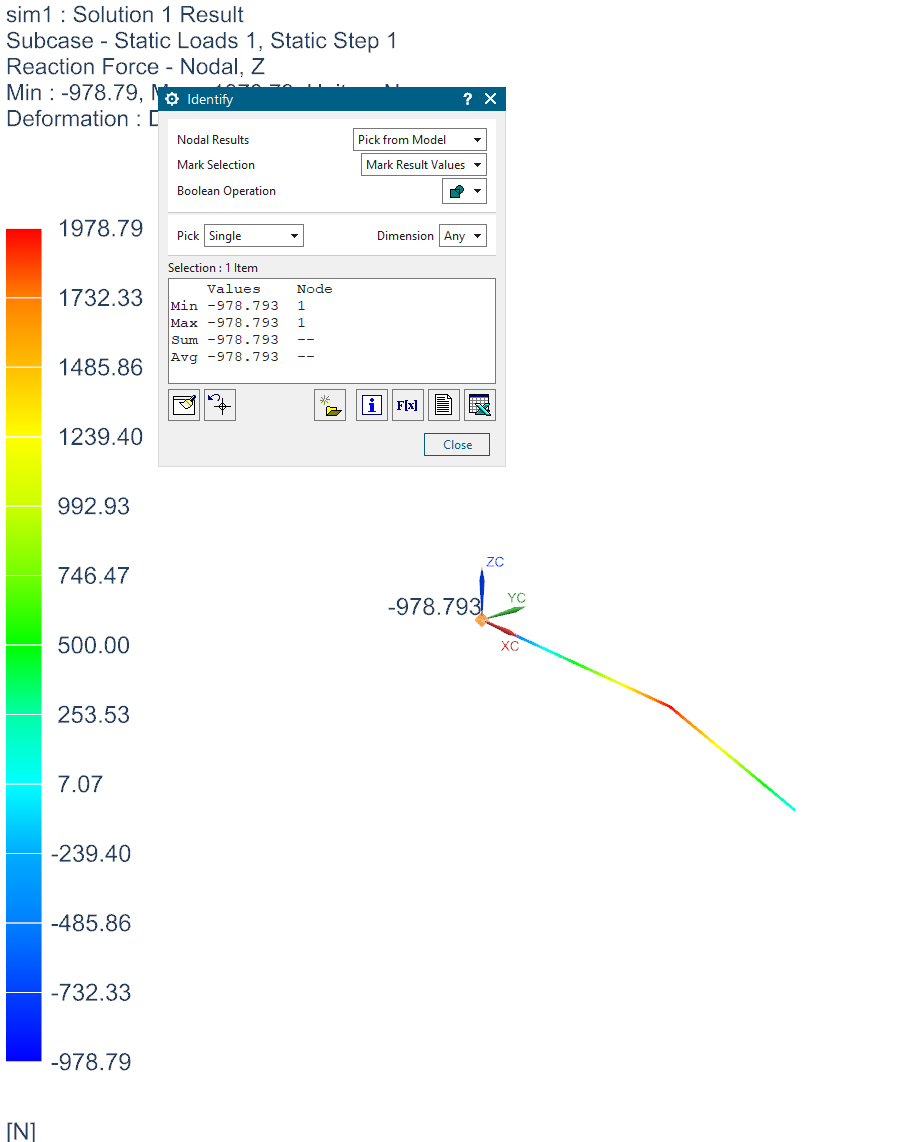BenjaminStarling
Subscriber
Again, there is nothing wrong. This is to be expected with FEM. The reaction force at the support on the right is 1000 N, the reaction on the left is 1000 N minus some error due to the coupling of strain to force. Beam packages usually are not solving partial derivatives, just a bunch of simultaneous equations that have pre-baked stiffness equations, this is why they yield perfect force results, and why they generally only do one type of analysis (piping, structural beams.. etc.) It is also why they generally cannot do transient analysis, as the mass term is not coupled, but can do Modal Superposition.nTwo things you can do to test this result.nChange the cross section to rectangular solid. Use 1 mm x 1000 mm, then run another analysis where it is 1000 mm x 1 mm.nSet the poissons ratio to 0. (or vary it numerous times and see the change in reaction force)nI ran the same simulation in Simcenter (NX Nastran) and get the same result for a 500x500mm cross section (978.793 (NX) vs. 979.01 (Ansys))nn